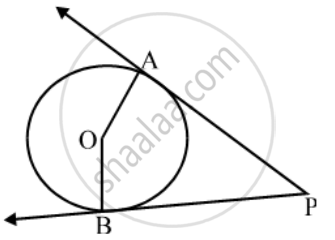# In fig., O is the centre of the circle, PA and PB are tangent segments. Show that the quadrilateral AOBP is cyclic. - Mathematics

Sum

In fig., O is the centre of the circle, PA and PB are tangent segments. Show that the quadrilateral AOBP is cyclic.

#### Solution

Since tangent at a point to a circle is perpendicular to the radius through the point.∴ OA ⊥ AP and OB ⊥ BP

⇒ ∠OAP = 90° and ∠OBP = 90°

⇒ ∠OAP +∠OBP = 90° + 90° ….(i)

∠OAP + ∠APB + ∠AOB + ∠OBP = 360°

⇒ (∠APB + ∠AOB) + (∠OAP +∠OBP) = 360°

⇒ ∠APB + ∠AOB + 180° = 360°

∠APB + ∠AOB = 180° ….(ii)

From equations (i) and (ii),we can say that the quadrilateral AOBP is cyclic.

Concept: Concept of Circle - Centre, Radius, Diameter, Arc, Sector, Chord, Segment, Semicircle, Circumference, Interior and Exterior, Concentric Circles
Is there an error in this question or solution?
Share